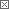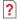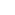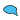Previous in Forum: 555 Timer To Control Motors Next in Forum: Differences & Similarities of SCADA Vs DCSAnonymous Poster

#How do Voltmeters Work?

03/25/2007 4:21 AM

how does the voltmeter measure the voltage drop or rise across a circuit.

Pathfinder Tags: circuit voltage drop voltmeters
Interested in this topic? By joining CR4 you can "subscribe" to
Power-User

Join Date: Feb 2007
Location: Oregon
Posts: 292#1

###Re: how does the voltmeter work

03/25/2007 4:24 PM

http://en.wikipedia.org/wiki/Voltmeter

Power-User

Join Date: Sep 2006
Posts: 367#2

###Re: How do Voltmeters Work?

03/26/2007 10:36 AM

Use two identical voltmeters.

First install/connect on the beginning of the circuit and second at the end.

Note voltage reading at the same time! The difference is the voltage drop.

Guru

Join Date: Dec 2006
Location: North America, Earth
Posts: 4395#3

###Re: How do Voltmeters Work?

03/27/2007 11:47 PM

It must draw some current out of the circuit, however so small. In a simple VOM the current develops a magnetic field that opposes a permanent magnet in the meter movement and causes a deflection upscale. Digital voltmeters can get quite complicated with dual-slope integation and so forth. They have higher input resistance, so draw very tiny currents out of the circuit.

S

__________________
“I would rather have questions that can't be answered than answers that can't be questioned.” - Richard Feynman
Active Contributor

Join Date: Oct 2006
Posts: 14#7

###Re: How do Voltmeters Work?

03/28/2007 9:26 AM

Digital voltmeters have most of the time an input resistance of 10 MegOhms.

Some analog voltmeters have an input resistance of 100,000 Ohms per Volt (DC only). Then mesuring a voltage of more than 100V dc, they derive less current than the digital models. This means their sensitivity full scale is 10 microAmps. Do'nt let them fall down: they are very fragile!

Power-User

Join Date: Sep 2006
Posts: 367#8

###Re: How do Voltmeters Work?

03/28/2007 2:00 PM

The DMM I have needs for its 0.4 V scale o.4 microAmp! (Because of 1 MegaOhm resistance).

Anonymous Poster#4

###Re: How do Voltmeters Work?

03/28/2007 4:59 AM

Voltage is the force on unit charge which is in the influence of electric field intensity.

By measuring voltage means to measure potential difference between two points.

One, placed in an electric field and the other at infinite distance.

WE MEASURE IT BY CONNECTING ONE TERMINALS OF DMM BETWEEN TWO TERMINALS.

Anonymous Poster#5

###Re: How do Voltmeters Work?

03/28/2007 5:04 AM

Voltage is the force on unit charge which is in the influence of electric field intensity.

By measuring voltage means to measure potential difference between two points.

One, placed in an electric field and the other at infinite distance.

WE MEASURE IT BY CONNECTING THE TERMINALS OF DMM BETWEEN TWO TERMINALS

Power-User

Join Date: Sep 2006
Posts: 367#6

###Re: How do Voltmeters Work?

03/28/2007 8:43 AM

It seems there are two questions:

1. How do Voltmeters work? (the physical application laws applied to their constructions)

2. How to measure voltage drop in the electrical circuit?

Each of the above posts answer this "student's test" questions.

Summary:

1. in 2007 two types of voltmeters are in use: Analog (as one of function of VOM = Volt - Ohm Meter) and Digital (Voltage ranges of DMM = Digital Multi-Meter). VOM in fact measure small current drawn from the circuit which is proportional to the voltage (difference of electric potentials); in the presence of the small permanent magnets this small currect creates torque to move the pointer on the VOM scale. The applied electromechanical law is the same as in electrical motors. The Pointer movement is stopped by spring. DMM measures voltage (voltage drop across the its input resistance); this small part of real/measured circuit voltage is amplified (by operational amplifier) and converted to the digital signal and displayed on digital display. DMM required a battery (as a auxiliary power to do the job), comparing to VOM that do move pointer taking energy from the circuit.

2. Voltage drop is the difference of two voltages in the power circuit, the one delivering electric power to the load. Here is applied Ohm's Law. An example:

Single phase AC (Alternating Current) receptacle has measured 120 Vac by DMM without a load. After plugging-in an electric heater of about 1200 W this voltage dropped to 108 V. So voltage drop (delta V) = 120 - 108 = 12V. You may estimate the circuits resistance: Load current is about I = 10 A, dV =12 V, R = dV/I = 12V/10A = 1.2 Ohm approx. This calculation is very rough because it did not respect several other factors as: resistance of heater change as temperature function, ignored inductance of the power circuit, etc. You need to take EET course for details... or do the measurements with all precautions to avoid damages to equipment or yourself!